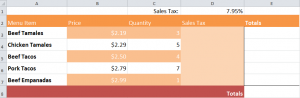# How to Use Cell References

6
JanExcel offers another function for users who facilitate the program for formula creation: Cell Reference Formulas. There are two types, Absolute and Relative. Learn how to use cell references through the example.

Download the example spreadsheet here.

## Absolute Cell Reference Formula:

For the Absolute formula, we will use Sales Tax calculation as the example. To retrieve the sales tax amount for each item in the spreadsheet, we need to multiply the price times the quantity (amount). After, we will take the answer and multiply it by the sales tax number in cell D1.

Put the following formula in the cell D3 to perform the calculation in this example: = (b3*c3)*\$d\$1

Using the fill handle function, drag the formula to other cells. The formula entered for B3 and C3 will change with each row’s own formula, but the Absolute reference to D1 (the sales tax) will always remain the same.

## Relative Cell Reference Formula:

In this formula, you can add the sales tax to the total for each individual item using Relative cell references.

In the example spreadsheet, go to the “totals” columns and add this formula to the first cell:

=(b3*c3)+d3

Referring to cells in this format – “B3“ – is known as Relative Referencing.

This formula will calculate the total for each of the items in their individual row. To complete this, click the fill handle tool and pull down the formula to cell E7.

The segment created from this action is called a range. To get the grand total in cell E8, input “=sum(e3:e7)” in the cell and you will have completed Cell References.